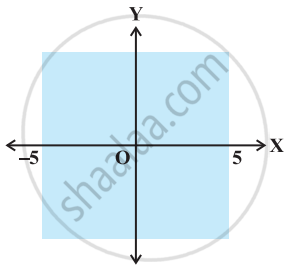# The inequality representing the following graph is ______. - Mathematics

MCQ
Fill in the Blanks

The inequality representing the following graph is ______.• |x| < 5

• |x| ≤ 5

• |x| > 5

• |x| ≥ 5

#### Solution

The inequality representing the following graph is |x| < 5.

Explanation:

The given graph represents x > –5 and x < 5.

Combining the two inequalities |x| < 5.

Concept: Algebraic Solutions of Linear Inequalities in One Variable and Their Graphical Representation
Is there an error in this question or solution?

#### APPEARS IN

NCERT Mathematics Exemplar Class 11
Chapter 6 Linear Inequalities
Exercise | Q 26 | Page 110
Share